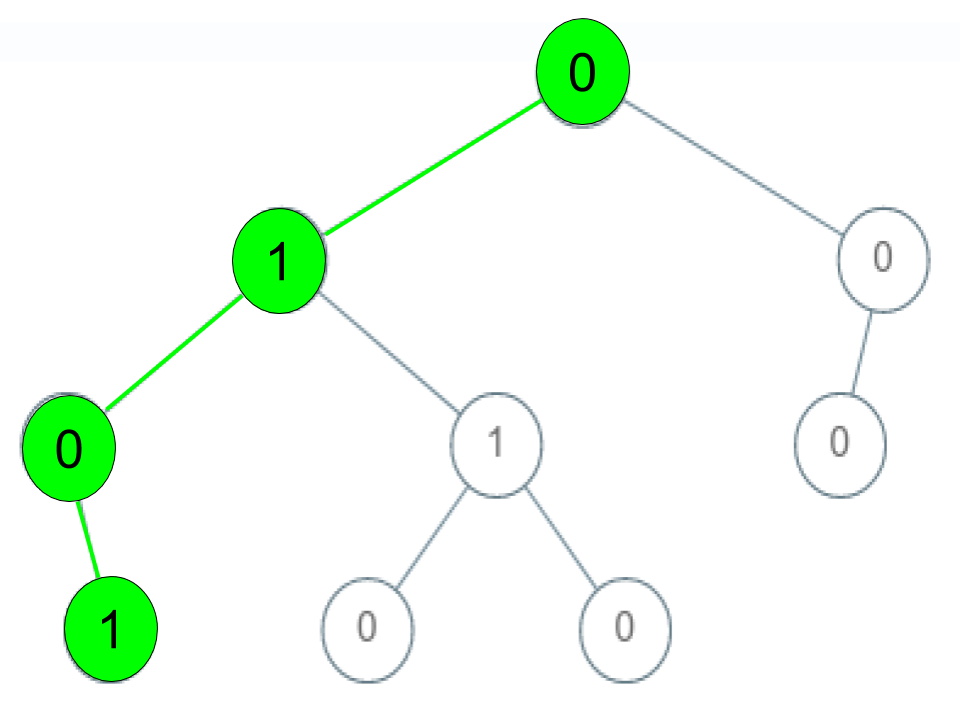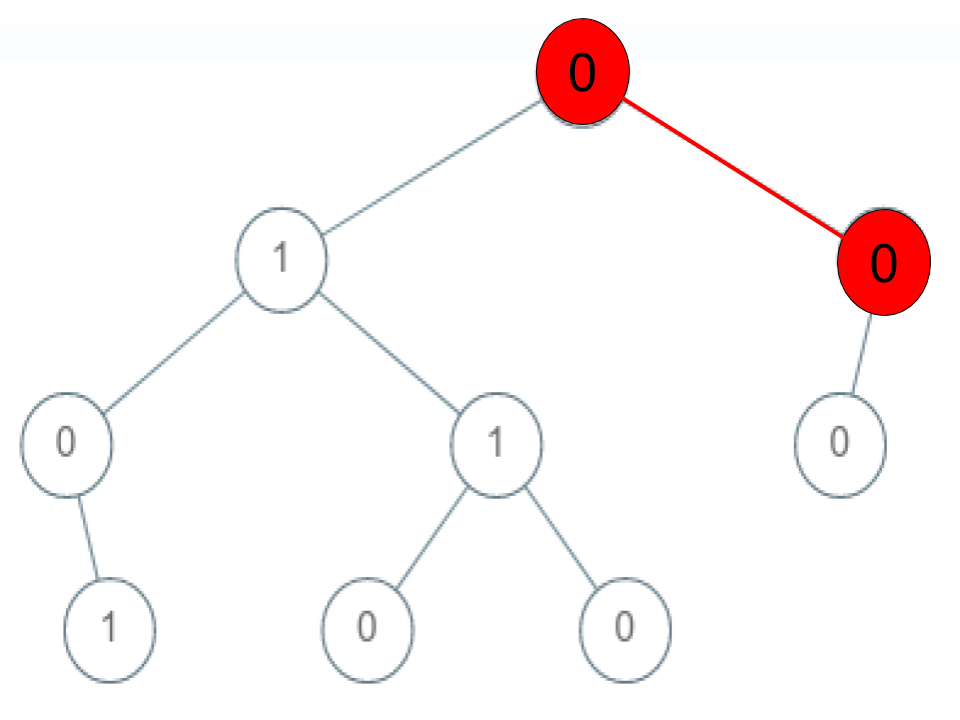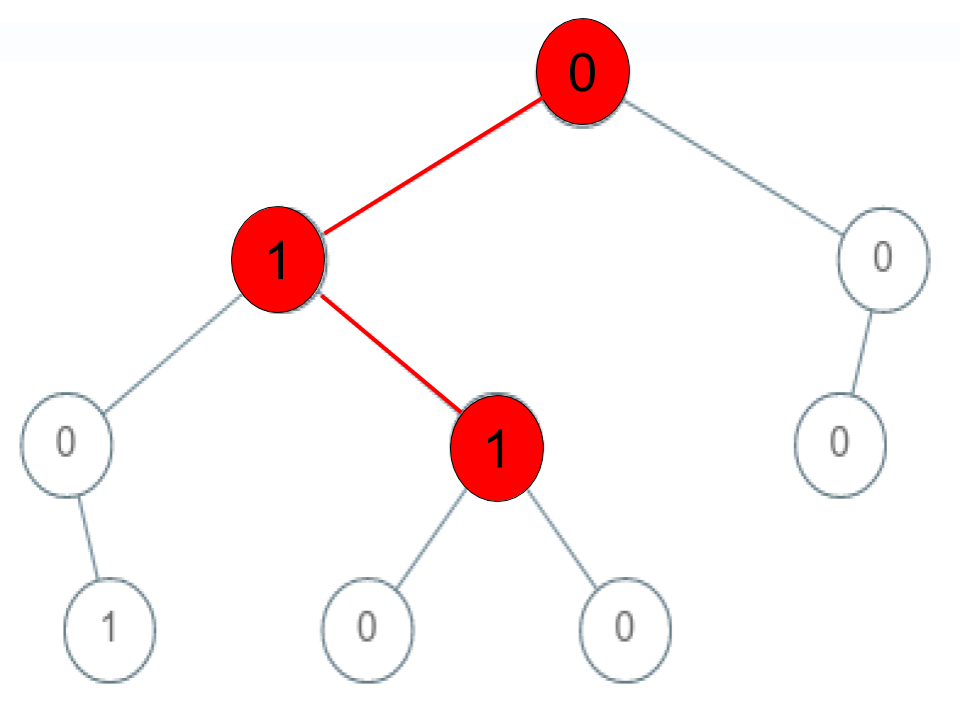Formatted question description: https://leetcode.ca/all/1430.html

# 1430. Check If a String Is a Valid Sequence from Root to Leaves Path in a Binary Tree (Medium)

Given a binary tree where each path going from the root to any leaf form a valid sequence, check if a given string is a valid sequence in such binary tree.

We get the given string from the concatenation of an array of integers arr and the concatenation of all values of the nodes along a path results in a sequence in the given binary tree.

Example 1:Input: root = [0,1,0,0,1,0,null,null,1,0,0], arr = [0,1,0,1]
Output: true
Explanation:
The path 0 -> 1 -> 0 -> 1 is a valid sequence (green color in the figure).
Other valid sequences are:
0 -> 1 -> 1 -> 0
0 -> 0 -> 0


Example 2:Input: root = [0,1,0,0,1,0,null,null,1,0,0], arr = [0,0,1]
Output: false
Explanation: The path 0 -> 0 -> 1 does not exist, therefore it is not even a sequence.


Example 3:Input: root = [0,1,0,0,1,0,null,null,1,0,0], arr = [0,1,1]
Output: false
Explanation: The path 0 -> 1 -> 1 is a sequence, but it is not a valid sequence.


Constraints:

• 1 <= arr.length <= 5000
• 0 <= arr[i] <= 9
• Each node's value is between [0 - 9].

## Solution 1.

// OJ: https://leetcode.com/problems/check-if-a-string-is-a-valid-sequence-from-root-to-leaves-path-in-a-binary-tree/

// Time: O(N)
// Space: O(H)
class Solution {
bool dfs(TreeNode* root, vector<int> &A, int i) {
if (i >= A.size()) return false;
if (!root || root->val != A[i]) return false;
if (i == A.size() - 1) return !root->left && !root->right;
return dfs(root->left, A, i + 1) || dfs(root->right, A, i + 1);
}
public:
bool isValidSequence(TreeNode* root, vector<int>& arr) {
if (!root) return arr.size() == 0;
return dfs(root, arr, 0);
}
};


Java

/**
* Definition for a binary tree node.
* public class TreeNode {
*     int val;
*     TreeNode left;
*     TreeNode right;
*     TreeNode() {}
*     TreeNode(int val) { this.val = val; }
*     TreeNode(int val, TreeNode left, TreeNode right) {
*         this.val = val;
*         this.left = left;
*         this.right = right;
*     }
* }
*/
class Solution {
public boolean isValidSequence(TreeNode root, int[] arr) {
if (root == null || root.val != arr)
return false;
int length = arr.length;
queue.offer(root);
int index = 0;
while (index < length && !queue.isEmpty()) {
int num = arr[index];
int size = queue.size();
for (int i = 0; i < size; i++) {
TreeNode node = queue.poll();
if (node.val == num) {
TreeNode left = node.left, right = node.right;
if (left != null)
queue.offer(left);
if (right != null)
queue.offer(right);
if (index == length - 1 && left == null && right == null)
return true;
}
}
index++;
}
return false;
}
}